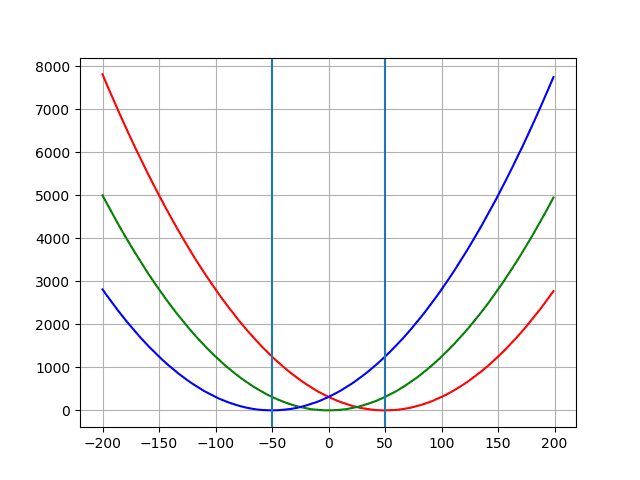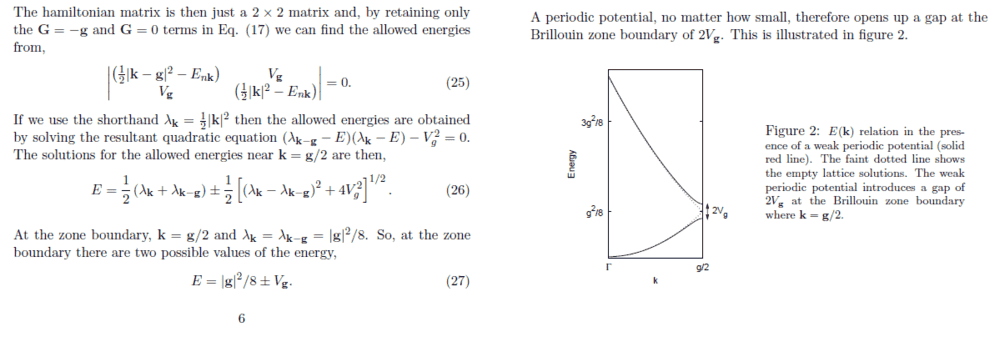# Plotting the solution of the central equation using python

patric44
Homework Statement:
the solution of the central equation in solids
Relevant Equations:
in the attachments
hi guys

i was trying to came up with a basic code that could show me the band gaps in a solid after adding the periodic potential term to my solution :
$$E = \frac{ħ^2q^2}{2m} \pm Vg$$
where Vg is my periodic potential , q is the k values in the first billion zone
from my understanding if i set the potential = 0 , i will get the normal parabolic dispersion for the free electron model , but once i introduce the potential it will create a separation at certain values of k which will indicate the forbidden gaps .
i tried to create that in python :
the plot of energy values with a periodic potential function:
from scipy import signal
import numpy as np
import matplotlib.pyplot as plt
import matplotlib.style
import matplotlib.colors
m = 1
ħ  = 1
G = 50
K = 200
E1 = []
E2 = []
E3 = []
x1 = []
x2 = []
x3 = []
def energy1(q):
erg1 = ((ħ**2 * (q-G)**2 )/(8*m))+ np.cos(q)
return erg1
def energy2(q):
erg2 = ((ħ**2 * q**2 )/(8*m))+ np.cos(q)
return erg2
def energy3(q):
erg3 = ((ħ**2 * (q+G)**2 )/(8*m))+ np.cos(q)
return erg3

for z in range(-K,K):
x1.append(z)
E1.append(energy1(z))
for z in range(-K,K):
x2.append(z)
E2.append(energy2(z))
for z in range(-K,K):
x3.append(z)
E3.append(energy3(z))
plt.plot(x1,E1,'r')
plt.plot(x1,E2,'g')
plt.plot(x1,E3,'b')
plt.axvline(x=G)
plt.axvline(x=-G)
plt.grid()
plt.show()

and it gives that :i was just trying to see the effect of basic periodic function like the sin , but there is no gaps! ,
i must be missing something or doing something wrong ?
any help will be appreciated , thanks

Fred Wright
Your model doesn't properly provide for how the periodicity of the lattice effects the electron wave function. Try studying the Kronig-Penny model, for example here, Electron in periodic lattice.

patric44
Your model doesn't properly provide for how the periodicity of the lattice effects the electron wave function. Try studying the Kronig-Penny model, for example here, Electron in periodic lattice.
i don't understand what you mean ? do you mean that the potential function that i picked will not give arise
to a band gap at the zone bounders , ( is it must be a square wave like the Kronig-Penny model ?!)
my solution was taken from this book :how would i modify this code to show the band gap at the zone boundaries ?

Fred Wright
how would i modify this code to show the band gap at the zone boundaries ?
In the link I provided the following dispersion relation is derived,$$\cos (ka)=\cosh (\alpha a)+P\frac{\sinh (\alpha a)}{\alpha a}\\ \alpha^2 =\frac{2m| E |}{\hbar^2}\\ P=\frac{mV_0 ba}{\hbar^2}$$where ##a## is the lattice constant, ##b## is the width of the well, and ##V_0## is the depth of the well. Your algorithm must pick an E value for the r.h.s. of the equation, then take the arccosine and divide by ##a## to determine the corresponding k. It's a two step process, choose ##E## then compute ##k##.

patric44
In the link I provided the following dispersion relation is derived,$$\cos (ka)=\cosh (\alpha a)+P\frac{\sinh (\alpha a)}{\alpha a}\\ \alpha^2 =\frac{2m| E |}{\hbar^2}\\ P=\frac{mV_0 ba}{\hbar^2}$$where ##a## is the lattice constant, ##b## is the width of the well, and ##V_0## is the depth of the well. Your algorithm must pick an E value for the r.h.s. of the equation, then take the arccosine and divide by ##a## to determine the corresponding k. It's a two step process, choose ##E## then compute ##k##.
thanks for helping , but i have a question :
why the paper from the book that i provided doesn't mention this , i mean the book just says
$$E=\frac{ħ^2q^2}{ 2m} \pm Vg$$
why the book didn't mention the kronig model , does the book has another approach or it was just being
very hand wavy about it ?!

Fred Wright
thanks for helping , but i have a question :
why the paper from the book that i provided doesn't mention this , i mean the book just says
$$E=\frac{ħ^2q^2}{ 2m} \pm Vg$$
why the book didn't mention the kronig model , does the book has another approach or it was just being
very hand wavy about it ?!
I don't have your book so I can't answer your question. I suspect that the chapter you are studying introduces Bloch's theorem but stops short of deriving a dispersion relation for a particular model...maybe in a subsequent chapter it will.